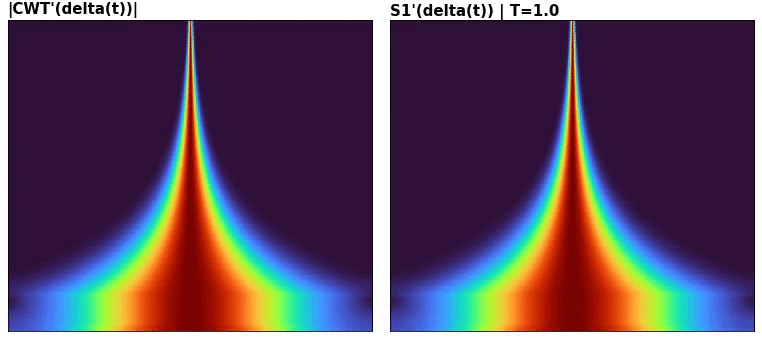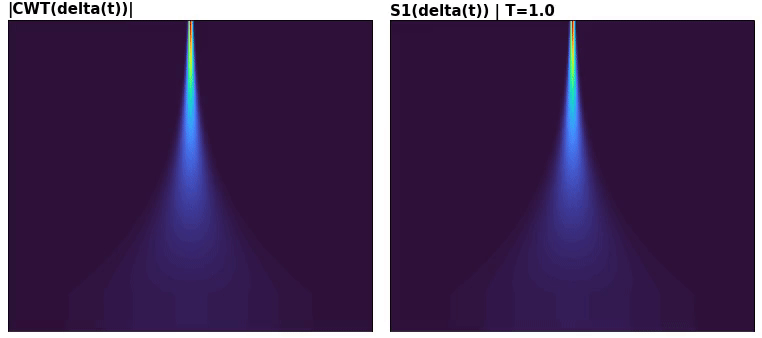# Wavelet Scattering time-warp equivariance

Is scattering equivariant to multiplicative time warps? Defined as

$$x(t) \rightarrow x(\tau(t)t),\ \text{sup}|\tau'(t)| < 1$$

This post claims it holds approximately - what are the arguments, and approximation conditions?

### Time-warp-frequency equivariance (multiplicative)

The argument is simple: CWT center frequencies are distributed exponentially. Adjacent coefficients are hence related multiplicatively in frequency: the very wavelets are multiplicatively warped. The only mismatch is that of temporal support, which also varies exponentially.

For exact equivariance we require

$$\text{supp}(x \star \psi_{\xi}) = \text{supp}(x \star \psi_{m\xi}), \ \forall m \in \mathbb{R} \tag{1}$$

where $$\text{supp}=$$ temporal support, and $$\xi$$ is some center frequency. This won't happen, as it's equivalent to fixing all wavelets' supports. Suppose a windowed signal with time support of 2, and wavelet $$\psi_\xi$$ of 10, and second wavelet $$\psi_{2\xi}$$:

• supp(conv(x, psi_xi )) = 2 + 10 = 12
• supp(conv(x, psi_2xi)) = 2 + 10*2 = 22

Note, if $$\text{supp}(x) \ggg \text{supp}(\psi)$$, then $$(1)$$ holds approximately:

• supp(conv(x, psi_xi )) = 1000 + 10 = 1010
• supp(conv(x, psi_2xi)) = 1000 + 10*2 = 1020

Alternatively, $$\text{supp}(\psi_{\xi_0}) \approx \text{supp}(\psi_{\xi_1})$$:

• supp(conv(x, psi_xi )) = 1000 + 10 = 1010
• supp(conv(x, psi_2xi)) = 1000 + 10*2 = 1020

### Equivariance vs commutativity

"Commutativity" is what we're really showing; the result is:

$$\text{warp}(\text{logfreq_shift}(x)) \Leftrightarrow \text{logfreq_shift}(\text{warp}(x))$$

It's "equivariance" in the sense of, warping has same effect on transform of a signal regardless of signal's location on time-frequency plane (the "time" part guaranteed by translation equivariance).

### Approximate commutativity: scattering

Last $$\text{supp}$$ result is key: scattering adds $$T$$ to effective support of every wavelet. Comparing impulse response$${}^1$$ of CWT and scattering, latter for all $$T$$; peak amplitude of each row has been normalized to be same for visual clarity (hence CWT', S'):(1: unlike usual, scattering's IR can't be used as surrogate in convolution (i.e. overlap-adding weighted IRs) due to modulus nonlinearity)

Comparing distant rows from CWT & scattering:### Example: echirp warp

$$x$$ is swept from $$128$$ to $$512\ \text{Hz}$$, $$y$$ from $$32$$ to $$128\ \text{Hz}$$, both warped with same $$|\tau(t)|$$. Instantaneous ridges:(Confirms the frequency ridges are exactly equivariant). CWT vs scattering:### Exact commutativity / Scale equivariance

There's commutativity in another sense: warping the signal can be traded for warping the wavelet, within a warp. Result from Lostanlen, sect 2.2.2):

Define "warp" as $$\mathcal{W}_{(a,b)}x(t) = a x(at + b)$$. Then,

\begin{align} (\mathcal{W}_{(a,b)}x \star \psi)(t) &= \int_{-\infty}^{\infty} x(t')\psi\left(t - \frac{t' - b}{a}\right) dt' \\ &= a\left(x \star \mathcal{W}_{(1/a, 0)}\psi) \vphantom{\frac{.}{.}} \right)(at + b) \\ &= \mathcal{W}_{(a, b)} \left(x \star \mathcal{W}_{(1/a, 0)}\psi \vphantom{\frac{.}{.}} \right)(t) \\ \end{align}

Verbally: filtering warped signal == warping warp-filtered signal. Illustratively,

• Suppose $$x$$'s and $$\psi$$'s peak frequencies match and are $$\xi$$. Then, LHS shifts $$x$$'s peak to $$a\xi$$, and $$\psi$$ multiplies with this shifted spectrum (in freq domain).
• RHS first shifts $$\psi$$'s peak to $$\xi/a$$; $$\psi$$ is now at same relative position to $$x$$ as in LHS, thus multiplies the same. The result is then shifted: $$\psi$$'s goes $$\xi/a \rightarrow \xi$$, and $$x$$'s goes $$\xi \rightarrow a\xi$$ - same as LHS.
• This won't work with STFT. While the peak shifts remain the same, the multiplication step fails due to differing relative bandwidth: fixed ratio of center frequency to bandwidth (CQT) is key.
• Put another way: all wavelets are warps of each other (in CQT).
• This does not prove the general $$\tau(t)$$ case, only affine.

Verifying temporal supports:

• LHS: 2 * 3 + 5 = 11
• RHS: 2 * (3 + 5/2) = 2 * 5.5 = 11

An equivalent verdict is much simpler - scale equivariance: scaling the input (in amplitude and time, i.e. "zooming"/"stretching") corresponds to scaling the output$${}^2$$. Hence, to state an equality, we undo this scaling with the inverse warp. It's a central motivation of wavelets - a "multi-scale zoom" (if we literally zoom on an image of a wavelet, it becomes another wavelet, scaled by the zoom factor). For scattering, this only holds above the scale of time-shift invariance, $$T$$ (see 'impulse response').

2: more precisely, corresponds to transforming the representation into the scaled region of the time-frequency plane. So a temporal stretching, and a log-frequency shift, such that new peak "resonant" wavelets are original ones scaled.

With steps:

\begin{align} (\mathcal{W}_{(a,b)}x \star \psi)(t) &= \int_{-\infty}^{\infty} a x(au + b)\psi(t - u) du \\ t' = au + b &\Rightarrow u = (t' - b)/a,\ dt'=adu \\ &\Rightarrow \int_{-\infty}^{\infty} x(t')\psi\left(t - \frac{t' - b}{a}\right) dt' \\ \end{align}

\begin{align} \left(x \star \mathcal{W}_{(1/a,0)}\psi \vphantom{\frac{.}{.}} \right)(t) & = \int_{-\infty}^{\infty} x(t - u) \frac{1}{a}\psi (u/a)du \\ t' = t - u \Rightarrow u = t - t',&\ dt' = -du,\ \infty \rightarrow -\infty \\ & \Rightarrow \frac{1}{a}\int_{-\infty}^{\infty} x(t') \psi ((t-t')/a)dt' \\ \mathcal{W}_{(a, b)}: t &\rightarrow at + b,\ \times a \\ & \Rightarrow \int_{-\infty}^{\infty} x(t') \psi ((at + b -t')/a)dt' \\ & = \int_{-\infty}^{\infty} x(t') \psi \left(t - \frac{t' - b}{a}\right)dt' \\ \end{align}

concludes the proof.

### Approximate commutativity (cont'd)

Scattering's contribution is more significant when realizing that CWT's scale equivariance includes amplitude scaling: $$\mathcal{W}_{(a,b)}x(t) = ax(at + b)$$. Scattering equalizes these as well; raw coefficients: